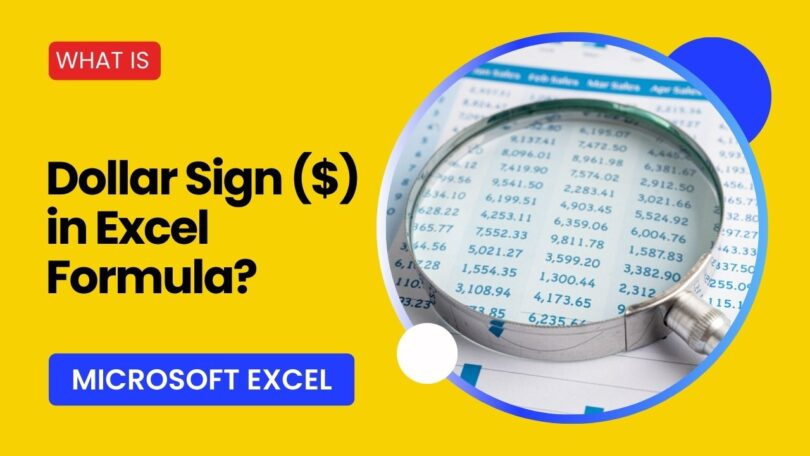Computer Science

# What is \$ in Excel Formula?Microsoft Excel is a powerful tool for data analysis, and its formulas are the backbone of many spreadsheet operations. One of the most intriguing yet often misunderstood aspects of Excel formulas is the use of the dollar sign (`\$`). This symbol is crucial in how Excel treats cell references when formulas are copied or moved.

In this article, we will delve into the significance of the dollar sign `\$` in Excel formula and how it can make your spreadsheet tasks more efficient.

## What is \$ in Excel Formula?

In MS Excel, the dollar sign `\$` is used for absolute referencing. When you use a dollar sign before the column letter and/or the row number, you’re telling Excel to keep that part of the reference constant when you copy the formula to another cell.

There are three types of cell references in Excel:

1. Relative Reference (`A1`)
2. Mixed Reference (`\$A1` or `A\$1`)
3. Absolute Reference (`\$A\$1`)

## Types of Cell References in Excel

Before we jump into the role of the dollar sign `\$`, it’s essential to understand the types of cell references in Excel:

1. Relative Reference: This is the most basic type of cell reference, where both the row and column references are relative. For example, if you have a formula in cell `B1` as `=A1`, and you copy this formula to the cell `B2`, the formula will automatically adjust to `=A2`.
2. Mixed Reference: In this type, either the column or the row is absolute (fixed), while the other is relative. For example, `\$A1` or `A\$1`.
3. Absolute Reference: Here, both the row and column references are absolute, meaning they will not change when the formula is copied to another cell. For example, `\$A\$1`.

Examples:

1. Relative Reference
Formula in `B1`: `=A1`
• If you copy this formula to `B2`, it will become `=A2`.
2. Mixed Reference
Formula in `B1`: `=\$A1`
• If you copy this formula to `B2`, it will still be `=\$A2`.
• If you copy this formula to `C1`, it will still be `=\$A1`.
3. Absolute Reference
Formula in `B1`: `=\$A\$1`
• If you copy this formula to any cell, it will always be `=\$A\$1`.

The dollar sign `\$` is particularly useful when copying and pasting formulas without changing specific cell references.

## The Role of the Dollar Sign (\$)

The dollar sign creates absolute and mixed references in Excel formulas. When you place a `\$` before the column letter or row number, that part of the reference becomes “locked” or “absolute,” meaning it will not change when the formula is copied or moved to another cell.

Examples:

1. Absolute Column and Row: `\$A\$1`
If you copy this formula to any other cell, the reference will remain `\$A\$1`.
2. Absolute Column, Relative Row: `\$A1`
If you copy this formula down a row, the column stays as `A`, but the row number will change.
3. Relative Column, Absolute Row: `A\$1`
If you copy this formula to the right, the row stays as `1`, but the column letter will change.

## Practical Applications of \$ in Excel

### 1. Budgeting and Financial Analysis

Suppose you have a fixed interest rate in a particular cell and want to use it in multiple calculations. By making the interest rate cell an absolute reference, you can copy the formula across various cells without changing the interest rate reference.

### 2. Data Lookup Functions

When using functions like `VLOOKUP` or `HLOOKUP`, you often need to specify a table array. Making this array an absolute reference ensures that the table array remains constant, even when the formula is copied to other cells.

### 3. Conversion Rates

If you’re working with currency or unit conversions, you can place the conversion rate in a single cell and use absolute referencing to apply the same rate across multiple calculations.

## Performance Considerations

While the dollar sign is incredibly useful, it’s essential to use it judiciously. Excessive use of absolute references can make your spreadsheet harder to manage and understand. Always consider whether a reference should be relative, mixed, or absolute based on the specific needs of your task.

## Conclusion

The dollar sign (\$) in Excel formulas is more than just a symbol; it’s a powerful feature allowing greater flexibility and accuracy in your calculations. Understanding how to use \$ in Excel effectively can save time and reduce spreadsheet errors. Whether you’re a beginner or an Excel veteran, mastering the dollar sign \$ will elevate your spreadsheet skills.